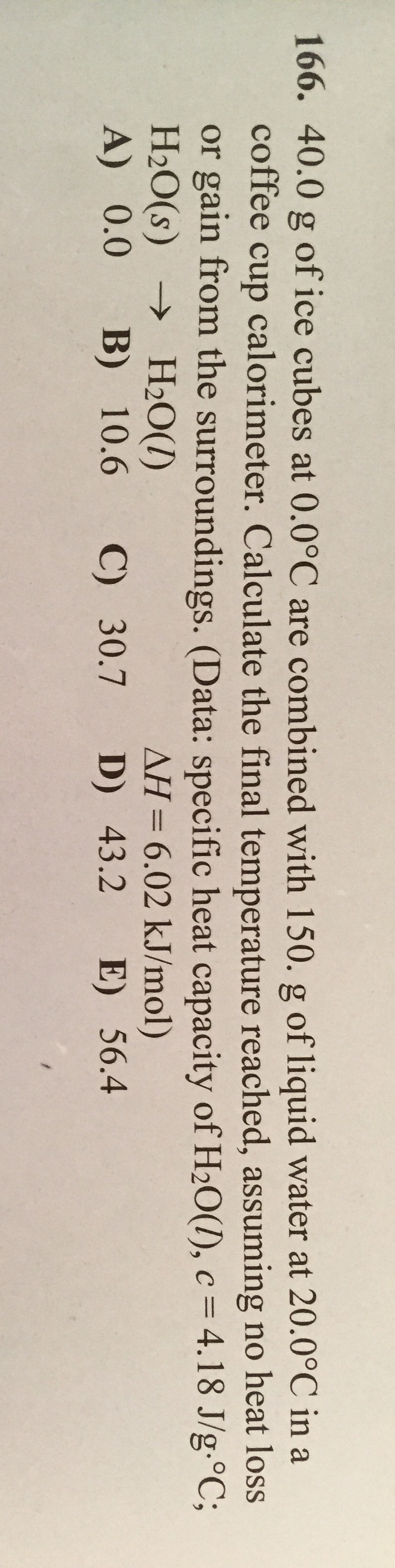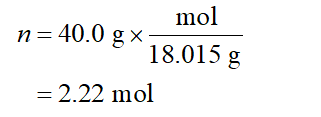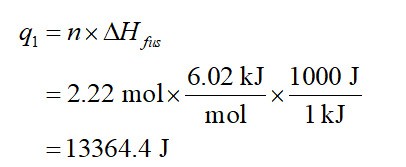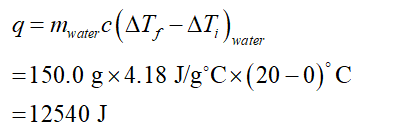# 166. 40.0 g of ice cubes at 0.0°C are combined with 150. g of liquid water at 20.0°C in acoffee cup calorimeter. Calculate the final temperature reached, assuming no heat lossor gain from the surroundings. (Data: specific heat capacity of H20(1), c = 4.18 J/g.°C;H2O(s) → H2O(1)A) 0.0%3DНаО()B) 10.6AH= 6.02 kJ/mol)C) 30.7 D) 43.2 E) 56.4%3D

Question
16 viewshelp_outlineImage Transcriptionclose166. 40.0 g of ice cubes at 0.0°C are combined with 150. g of liquid water at 20.0°C in a coffee cup calorimeter. Calculate the final temperature reached, assuming no heat loss or gain from the surroundings. (Data: specific heat capacity of H20(1), c = 4.18 J/g.°C; H2O(s) → H2O(1) A) 0.0 %3D НаО() B) 10.6 AH= 6.02 kJ/mol) C) 30.7 D) 43.2 E) 56.4 %3D fullscreen
check_circle

Step 1

The molar mass of water is 18.015 g/mol. The number of moles of water in the 40.0 g sample is calculated below.Step 2

The heat needed to change from solid at 0C to liquid at 0C is calculated below.Step 3

The heat lost by water is calculated below....

### Want to see the full answer?

See Solution

#### Want to see this answer and more?

Solutions are written by subject experts who are available 24/7. Questions are typically answered within 1 hour.*

See Solution
*Response times may vary by subject and question.
Tagged in